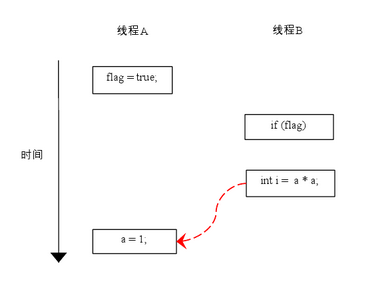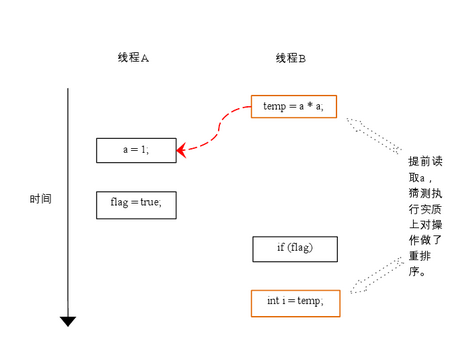# 1 线程安全

## 1.1 案例

``````public class ThreadTrain implements Runnable {
private int trainCount = 100;
@Override
public void run() {
while (trainCount > 0) {
try {
} catch (Exception e) {
}
sale();
}
}
public void sale() {
if (trainCount > 0) {
trainCount--;
}
}
public static void main(String[] args) {
t1.start();
t2.start();
}
}
``````

## 1.2 多线程之间线程安全问题

• 如何解决多线程之间线程安全问题
答:使用多线程之间同步synchronized或使用锁(lock)。
• 为什么使用线程同步或使用锁能解决线程安全问题呢？
答:将可能会发生数据冲突问题(线程不安全问题)，只能让当前一个线程进行执行。代码执行完成后释放锁，让后才能让其他线程进行执行。这样的话就可以解决线程不安全问题。
• 什么是多线程之间同步
答:当多个线程共享同一个资源,不会受到其他线程的干扰。

# 2 内置锁synchronized

Java提供了一种内置的锁机制来支持原子性

• 修饰需要进行同步的方法（所有访问状态变量的方法都必须进行同步），此时充当锁的对象为调用同步方法的对象
• 同步代码块和直接使用synchronized修饰需要同步的方法是一样的，但是锁的粒度可以更细，并且充当锁的对象不一定是this，也可以是其它对象，所以使用起来更加灵活

## 2.1 同步代码块synchronized

synchronized实现原理：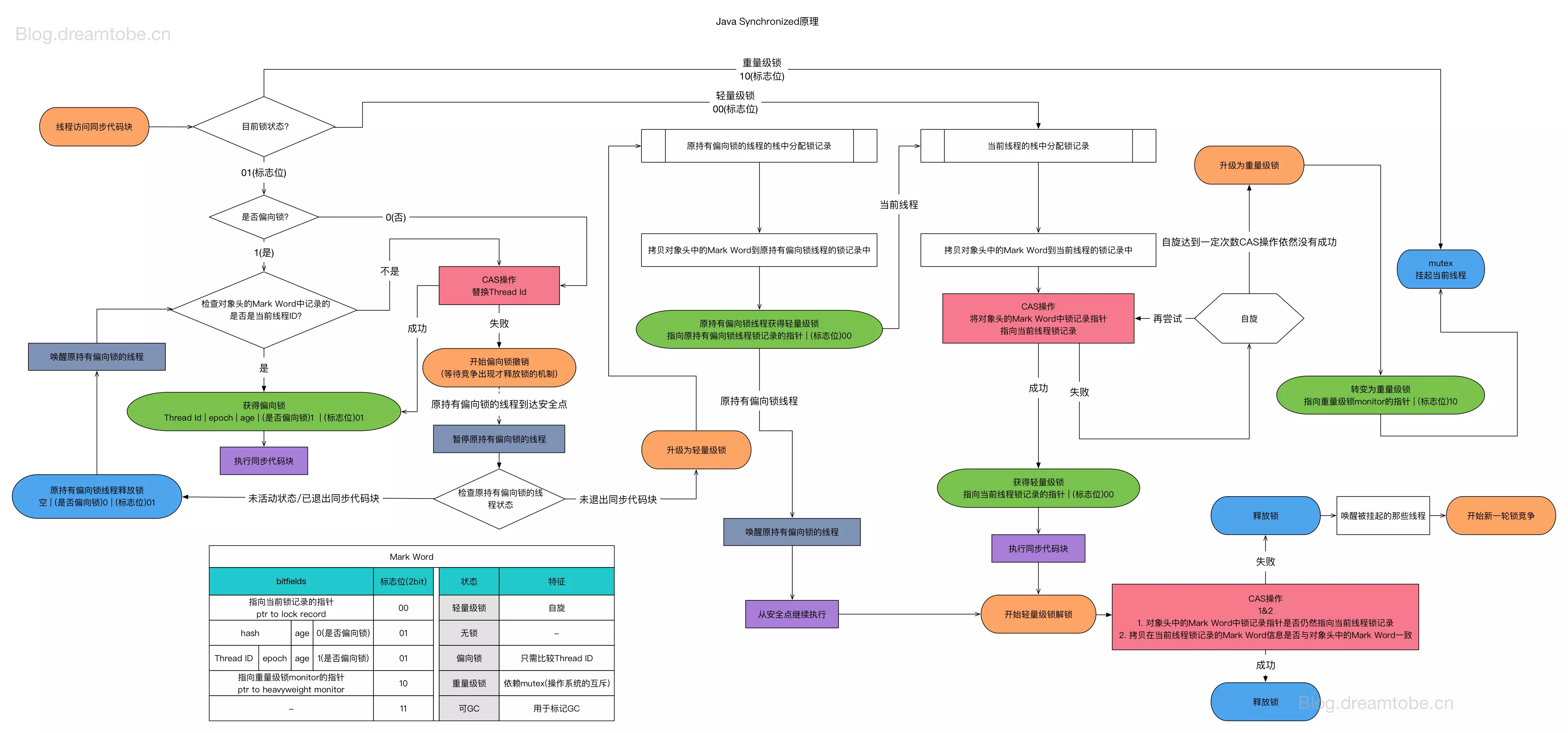• 必须要有两个或者两个以上的线程
• 必须是多个线程使用同一个锁
• 必须保证同步中只能有一个线程在运行

``````public void sale() {
synchronized (this) {
if (trainCount > 0) {
trainCount--;
}
}
}
``````

## 2.2 同步方法

``````public synchronized void sale() {
if (trainCount > 0) {
trainCount--;
}
}
``````

## 2.3 同步方法使用的是什么锁？

``````class Thread009 implements Runnable {
private int trainCount = 100;
private Object oj = new Object();
public boolean flag = true;
public void run() {
if (flag) {
while (trainCount > 0) {
synchronized (this) {
try {
} catch (Exception e) {
// TODO: handle exception
}
if (trainCount > 0) {
System.out
.println(Thread.currentThread().getName() + "," + "出售第" + (100 - trainCount + 1) + "票");
trainCount--;
}
}
}
} else {
while (trainCount > 0) {
sale();
}
}
}
public synchronized void sale() {
try {
} catch (Exception e) {
// TODO: handle exception
}
if (trainCount > 0) {
System.out.println(Thread.currentThread().getName() + "," + "出售第" + (100 - trainCount + 1) + "票");
trainCount--;
}
}
}
public class Test009 {
public static void main(String[] args) throws InterruptedException {
t1.start();
t2.start();
}
}
``````

## 2.3 静态同步函数

``````public static void sale() {
if (trainCount > 0) {
trainCount--;
}
}
}
``````

synchronized 修饰方法使用锁是当前this锁。
synchronized 修饰静态方法使用锁是当前类的字节码文件

# 3 多线程死锁

``````class Thread009 implements Runnable {
private int trainCount = 100;
private Object oj = new Object();
public boolean flag = true;
public void run() {
if (flag) {
while (trainCount > 0) {
synchronized (oj) {
try {
} catch (Exception e) {
// TODO: handle exception
}
sale();
}
}
} else {
while (trainCount > 0) {
sale();
}
}
}
public synchronized void sale() {
synchronized (oj) {
try {
} catch (Exception e) {
}
if (trainCount > 0) {
System.out.println(Thread.currentThread().getName() + "," + "出售第" + (100 - trainCount + 1) + "票");
trainCount--;
}
}
}
}
public class Test009 {
public static void main(String[] args) throws InterruptedException {
t1.start();
t2.start();
}
}}
``````

void set(Object value)设置当前线程的线程局部变量的值
public Object get()该方法返回当前线程所对应的线程局部变量
public void remove()将当前线程局部变量的值删除，目的是为了减少内存的占用
protected Object initialValue()返回该线程局部变量的初始值，这个方法是一个延迟调用方法，在线程第1次调用get()或set(Object)时才执行，并且仅执行1次

``````class Res {
// 生成序列号共享变量
public static Integer count = 0;
protected Integer initialValue() {
return 0;
};
};
public Integer getNum() {
int count = threadLocal.get() + 1;
return count;
}
}
private Res res;
this.res = res;
}
@Override
public void run() {
for (int i = 0; i < 3; i++) {
}
}
public static void main(String[] args) {
Res res = new Res();
}
}
``````

Map.put(“当前线程”,值)；

# 5 多线程三大特性

## 5.3 有序性

int a = 10; //语句1
int r = 2; //语句2
a = a + 3; //语句3
r = a*a; //语句4

# 6 Java内存模型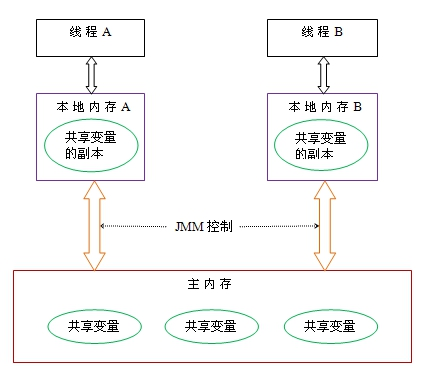• 首先，线程A把本地内存A中更新过的共享变量刷新到主内存中去。
• 然后，线程B到主内存中去读取线程A之前已更新过的共享变量。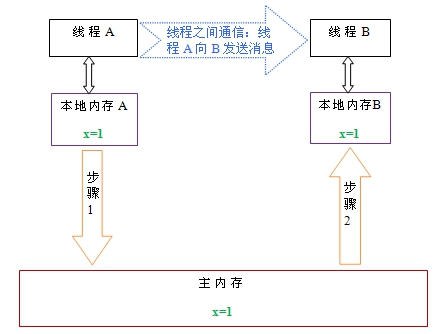# 7 volatile

## 7.1 volatile含义

Volatile保证了线程间共享变量的及时可见性，但不能保证原子性

``````class ThreadVolatileDemo extends Thread {
public    boolean flag = true;
@Override
public void run() {
System.out.println("开始执行子线程....");
while (flag) {
}
System.out.println("线程停止");
}
public void setRuning(boolean flag) {
this.flag = flag;
}
}
public static void main(String[] args) throws InterruptedException {
System.out.println("flag 已经设置成false");
}
}
``````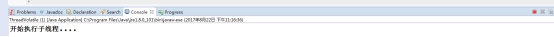** 原因:线程之间是不可见的，读取的是副本，没有及时读取到主内存结果。

## 7.2 volatile特性

• 保证此变量对所有的线程的可见性，这里的“可见性”，如本文开头所述，当一个线程修改了这个变量的值，volatile 保证了新值能立即同步到主内存，以及每次使用前立即从主内存刷新。但普通变量做不到这点，+普通变量的值在线程间传递均需要通过主内存（详见：Java内存模型）来完成。

## 7.3 volatile 性能

volatile 的读性能消耗与普通变量几乎相同，但是写操作稍慢，因为它需要在本地代码中插入许多内存屏障指令来保证处理器不发生乱序执行。

## 7.4 volatile与Synchronized区别

• 从而我们可以看出volatile虽然具有可见性但是并不能保证原子性。
• 性能方面，synchronized关键字是防止多个线程同时执行一段代码，就会影响程序执行效率，而volatile关键字在某些情况下性能要优于synchronized。
• 但是要注意volatile关键字是无法替代synchronized关键字的，因为volatile关键字无法保证操作的原子性

# 8 重排序

## 8.2 as-if-serial语义

``````double pi  = 3.14;    //A
double r   = 1.0;     //B
double area = pi * r * r; //C
``````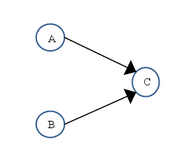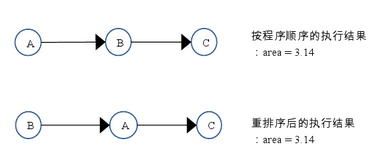as-if-serial语义把单线程程序保护了起来，遵守as-if-serial语义的编译器，runtime 和处理器共同为编写单线程程序的程序员创建了一个幻觉：单线程程序是按程序的顺序来执行的。as-if-serial语义使单线程程序员无需担心重排序会干扰他们，也无需担心内存可见性问题。

## 8.3 程序顺序规则

1. A happens- before B；
2. B happens- before C；
3. A happens- before C；

## 8.4 重排序对多线程的影响

``````class ReorderExample {
int a = 0;
boolean flag = false;
public void writer() {
a = 1;                   //1
flag = true;             //2
}
if (flag) {                //3
int i =  a * a;        //4
……
}
}
}
``````